# Performance Analysis of the Distance Relay Characteristics in a Compensated Transmission Line

Performance Analysis of the Distance Relay Characteristics in a Compensated Transmission Line
The Department of Electrical Engineering, College of Engineering, Mutah University, Karak 61621, Jordan

Electrical Power Engineering Department, Hijjawi Faculty for Engineering, Yarmouk University, Irbid 21163, Jordan

Corresponding Author Email:
Page:
197-205
|
DOI:
https://doi.org/10.18280/ejee.230304
2 November 2020
|
Accepted:
18 April 2021
|
Published:
21 June 2021
| Citation

OPEN ACCESS

Abstract:

The present work investigates the effect of the FACTS devices on distance relay operation. FACTS devices have different advantages in power system performance, stability, and load ability. In this paper, FACTS technologies' effect on the distance protective relay is presented using the measured impedance between the fault location and the relaying point. Different factors and parameters are changed to see their impacts on the studied system. It is shown that the measured impedance is affected by the presence of the FACTS devices depending on its type (series, parallel, and hybrid), fault location, and the operation point of the FACTS device. The analyses present that the shunt FACTS devices' effect may cause overreach problem to the relay; however, series FACTS devices may cause under reach problems in distance characteristics. MATLAB 2019b does the simulation test; the results of the simulation proved the mathematical analysis. The numerical analysis in this paper may be used for researchers in fault analysis and protection coordinators.

Keywords:

distance protection, FACTS devices, MATLAB, measured impedance

1. Introduction

Fixable AC transmission system (FACTS) devices are widely used in high voltage transmission systems. The benefits of using FACTS, such as load flow, economic benefits and stability enhancement, make it very powerful and desirable in the system. FACTS devices as a power flow controller based on power electronics technologies divide into two main categories; thyristor-based technology such as thyristor-controlled series capacitor (TCSC), static var compensator (SVC), thyristor-controlled voltage regulator (TCVR), and thyristor-controlled phase angle regulator (TCPAR); and voltage source converter-based technologies such as static synchronous series compensator (SSSC), static VAR system (SVS), static synchronous compensator (STATCOM), unified power flow controller (UPFC). Each device should be connected in series or parallel with the transmission line to inject voltage or current to the system. So, the protective relay will see the device as impedance during the fault. Series compensating dives, where the capacitors are connected in series with the transmission line, are used mainly to increase transfer capability. In contrast, the shunt devices are installed to improve the voltage profile and reduce line losses.

Different researchers analyze the performance of the distance relay in compensated transmission lines. In Ref. , detailed discussions on the distance relay principle and compare the performance of distance relay with and without FACTS devices are presented. The simulation of SSSC, STATCOM, and UPFC effect on measured impedance is shown. As a result, the measured resistance for both UPFC and STATCOM starts from a high value. However, a single-phase to ground fault is mentioned without detailed analysis based on the sequence component. Other researchers  present the effect of STATCOM on the performance of the distance relay. A real-time digital simulator-based hardware in the loop is established in this research. Other studies [3-7] show the effect of STATCOM on distance relay under different system configurations, fault locations, and fault conditions. The impacts of SSSC on measured impedance at the relay point for various fault locations are discussed in Refs. [8-10]. New techniques are presented in the literature to protect the transmission line may be affected in the compensated lines [11, 12]. The performance of digital relay in UPFC compensated transmission line is presented in literatures [13-15]. In these researches the effect of UPFC on measured impedance, which results in tripping boundaries of distance relay, is shown.

This paper provides a comprehensive analysis of the measured impedance on compensated transmission lines for different fault types, fault locations, system operations, and various FACTS devices. The rest of this paper is presented as follows: section (II) shows a summary of the FACTS devices types and their process. Different types of FACTS devices are presented. In section (III), the mathematical analysis of measured impedance at different fault types and different FACTS devices is presented. The characteristic of the distance relay and proposed pilot scheme to compensated transmission lines are presented in section (IV). The simulation results of the proposed model built on MATLAB 2019b /SIMULINK is shown in the Vth section, and the conclusion is shown in section (VI).

2. Facts Devices Model

There are mainly three types of FACTS devices from the view of its connection; series, parallel, and hybrid device. SSSC, SVC, and UPFC are examples of series, parallel and hybrid type FACTS devices, respectively. These devices are installed at the transmission line in order to enhance voltage profile, economic dispatch, maximize load ability, minimize total error etc. Moreover, the power flow of any transmission line is given by:

$S=V_{s}\left(\frac{V_{s}-V_{r}}{Z_{l}}\right)^{*}$     (1)

where, $V_{s}$, $V_{r}$: sending and receiving end complex voltages respectively, $Z_{l}$: line impedance. * indicates the conjugate operator. From this equation, the real and reactive power can be written as:

$P=\frac{v_{s} v_{r}}{X_{l}} \sin \delta$     (1a)

$Q=\frac{v_{s}\left(v_{s}-v_{r} \cos \delta\right)}{X_{l}}$     (1b)

where, $v_{s}$, $v_{r}$: voltage magnitude of sending and receiving end of the transmission lines. $\delta$: power angle the difference angle between the voltages at the terms of the line. Based on these equations, FACTS devices can be categorized as follows :

• Voltage regulators: change the magnitude of the voltage at the sending end to control both real and reactive power flow. e.g., STATCOM, SVC, TCVR, SVS-based voltage regulator. These controllers should have a shunt part to inject current (so reactive power) to the system.
• Line impedance compensators: induce a controlled capacitance or inductance in series with the line inductance. e.g., TCSC, SSSC. These devices should have a series part to inject voltage out of phase to the line current by ±90°.
• Phase angle regulation (Phase shift): these FACTS devices change the voltage's angle, but the magnitude does not change.

Unified power flow controller (UPFC): this particular configuration may change all line parameters to independently control both real and reactive power. This configuration has series and shunts VSC connected via a DC link (capacitor).

3. Mathematical Analysis

3.1 Three phase faults

For the analysis purposes, the system in Figure 1 is used. The test system consists of two strong sources (source A and source B) connected via a transmission line and two stations. In the case of UPFC disconnected, simply the balance impedance seen by relay R is given by Eq. (2) and Eq. (2a).

$\mathrm{Z}_{\mathrm{R}}=\mathrm{m} \mathrm{Z}_{\mathrm{L}}$      (2)

$Z=\frac{V_{a}}{I_{a}}$ or $\frac{V_{b}}{I_{b}}$ or $\frac{V_{c}}{I_{c}}$     (2a)

where, $\mathrm{Z}_{\mathrm{R}}$: impedance seen by the relay R from its Current transformer (CT) position to the fault location without the FACTS device (actual value). m: a factor of the line which represent the location of the fault to the relay. From Figure 1, the index m could be written as:

$m=\frac{Z_{L A}}{Z_{L A}+Z_{L B}}$     (2b)

where, $Z_{L A}$: impedance between the relay position and the fault location. The series $\left(Z_{S E}\right)$ and shunt $\left(Z_{s h}\right)$ FACTS impedances refer to the line impedance can represent as following relations:

## 1.png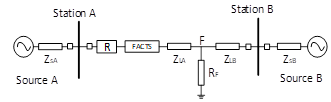Figure 1. Two machines test system with FACTS located at station A

$k_{s}=\frac{z_{S E}}{Z_{L}}$     (3a)

$k_{h}=\frac{Z_{S h}}{Z_{L}}$      (3b)

The measured impedance by the relay $\left(Z_{R}{ }^{\prime}\right)$ for the compensated transmission line is affected by $K_{s}$ and $K_{h} .$ The relation between measured impedance in the present of FACTS device and without FACTS device in term of $K_{s}$ and $K_{h}$ can be found as follows:

$E F=\frac{Z_{R}^{\prime}}{Z_{R}}=\frac{\left(m+K_{s}\right)\left(\frac{K_{h}}{m}\right)}{m+K_{S}+K_{h}}$       (4)

EF is called Error Factor. Eq. (4) presents the impact of $K_{s}$ and $K_{h}$ on measured impedance by distance relay when a fault occurred behind the FACTS device. If the fault occurs between the FACTS device and relay, the relay will work correctly.

3.1.1 Series FACTS device

The series FACTS device may consider as a particular operation point of UPFC when $K_{h} \rightarrow \infty$. The error factor of the series FACTS device ($E F_{S}$) is given as folow:

$E F_{s}=\left(1+\frac{k_{s}}{m}\right)$     (5)

$\left|E F_{s}\right|=\sqrt{1+\frac{\left|k_{s}\right|^{2}}{m^{2}}+\frac{2\left|k_{s}\right|}{m} \cos \theta_{s}}$      (5a)

$\theta_{\mathrm{EF} . \mathrm{s}}=\tan ^{-1}\left[\frac{\left|k_{s}\right| \sin \theta_{s}}{m+\left|k_{s}\right| \cos \theta_{s}}\right]$      (5b)

where, $\theta_{s}:$ the angle of the series factor $\left(k_{s}\right) \cdot \theta_{\mathrm{EF.} .}$ : the angle of the error factor (EF). From this relation, the effect of series FACTS devices on distance relay can be determined. Three parameters play significant impacts on this relation; $K_{s}, \theta_{s}$, and $\mathrm{m}$.

## 2.png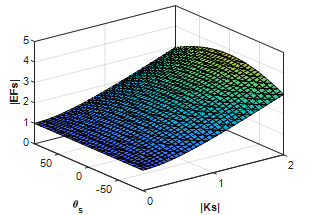Figure 2. The impact of the factor Ks on the magnitude of the measured impedance

## 3.png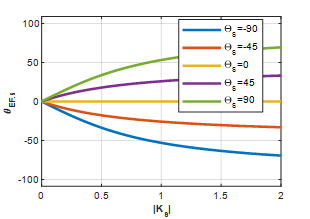Figure 3. The impact of the factor Ks on the angle of the measured impedance

The first zone of the distance relay is the most critical issue because the FACTS device protection will isolate the device before the set time of the second zone is gone. So, m = 0.75, which refers to the first zone fault, will be considered here. Figures 2 and 3 show the impact of series FACTS parameters on the measured impedance's magnitude and angle, respectively.

To avoid the effect of the series FACTS device on the distance operation, the controller should send the operation point ($K_{s}$) to the relay then the relay should make the proper correction to find the actual impedance. Some of the series of FACTS devices inject reactance to the line without resistance. In this case, the angle between line impedance (~70°) and the injected reactance is in the range of (~20°) in case of an inductive operation case and about (-160°) in case of a capacitive operation case.

The effect of this type of FACTS device (which inject inductance in series) can be ignored if the magnitude of $K_{s}$  greater than one by adding about 15° to the measured impedance. Otherwise, the relay should know the device's operation point to make a proper correction on the measured impedance.

From Figure 2, it can be noted that EF's value is higher than one in most cases. That means the series of FACTS devices make a problem of underreach in most operation cases, but this note depends on the operation case of the device. From Figure 2, it can say that the measured impedance is less than actual when $K_{s}<1.5$ and $\theta_{s}$ around 180° or -180°, capacitance case; otherwise, the measured impedance is higher than the actual. Based on these notes, we can generalize the following comments. If the FACTS device consumes reactive power (inductance case), the relay may see the fault impedance higher than the actual (under reach). If the FACTS device operation is leading (capacitive case) the relay may see the fault impedance lower than the real (overreach). From this observation, if the relay knows the FACTS device's setting point, it will be able to see when the device will operate lagging or leading based on measured power at the relay point.

For the MHO characteristic or impedance-direction characteristic relay, the small deviation in measured angle may not impact the relay operation. Based on this comment, the magnitude of impedance may be corrected with ignored the variation in the angle based on measured power and predefined setting point of the FACTS device. Some suitable operation cases can be mentioned here; as an example, the effect of the series part of UPFC can neglect if Kse = 0.5 and θs around 100° or 260°, as shown in Figure 2. These operation points may be found from Eq. (5a) as follow:

$m=\frac{Z_{L A}}{Z_{L A}+Z_{L B}}$     (5c)

At the summary of this section, some recommended notes for operators or protection coordinators can be summarized as follow:

• If $|K s|$ controlled and θs select to be constant, the magnitude of EF will be very sensitive to Ks, and the angle of $\theta_{E F}$ is almost lag θs by (0-30°) if Ks less than 0.5 (Figure 3). This note regards line impedance compensators and some particular operations of the UPFC.
• If θs controlled and Ks select to be constant, the magnitude of EF will be very sensitive to θs, the angle of $\theta_{E F}$ is almost lag θs by around 15°. This note regards phase angle regulators and particular operation points of the UPFC.

3.1.2 Shunt FACTS device

From Eq. (4), if we ignore the series part of the FACTS device ($K_{s}=0$) the error factor of the shunt FACTS device can be written as:

$E F_{h}=\frac{K_{h}}{m+K_{h}}$     (6)

$\left|E F_{h}\right|=\sqrt{\frac{\left|k_{h}^{2}\right|}{m^{2}+2 m\left|k_{h}\right| \cos \theta_{h}+\left|k_{h}\right|^{2}}}$      (6a)

$\theta_{\mathrm{EF} \cdot \mathrm{h}}=\theta_{\mathrm{h}}-\tan ^{-1}\left[\frac{\left|k_{h}\right| \sin \theta_{h}}{m+\left|k_{h}\right| \cos \theta_{h}}\right]$      (6b)

where, $\theta_{h}$: the angle of the shunt factor. Eq. (6a) shows the effect of $\left|K_{h}\right|, \theta_{h}$, and $\mathrm{m}$ on the magnitude of $E F_{h}$. Figures 4-5 show the shunt FACTS device's effect on the magnitude and the angle of the measured impedance at m=0.75, respectively. Figure 4 shows that the measured impedance is lower than the actual for both lag and lead operation points. Increasing the value of the magnitude of the shunt factor decreases the effect on the measured impedance.

## 4.pngFigure 4. The effect of the factor Kh on the magnitude of the measured impedance

## 5.pngFigure 5. The effect of the factor Kh on the angle of the measured impedance

The effect of the Shunt device on the angle of the measured impedance is shown in Figure 5. From the figure, it can be observed that the impact of the shunt device on the measured impedance's angle may be neglected if the $\theta_{h}$ around zero or Kh greater than 5. On the other hand, the effect of the shunt FACTS devices on the magnitude of the measured impedance can be neglected if Kh > 5 (Figure 4).

For the UPFC, shunt and series parts are operated independently, from the controller viewpoint; therefore, the shunt part can make a control on the reactive power flow (so voltage magnitude), and the series part can make a control on the real power flow. Thus, the voltage magnitude, the voltage angle, and the line's impedance can be changed independently by the UPFC. Two of them are enough to regulate the real and the reactive power flow in the line based on Eq. (1a) and Eq. (1b). So, if the UPFC is used only to hold the power flow (real and imaginary), it will have one free parameter. Based on this additional freedom in the control axis, it is possible to operate the UPFC in a lower impact on the distance relay. If the factor $\mathrm{EF}=1 \angle 0$ the relay will not be affected by the existence of the UPFC. So, from Eq. (4), this condition can achieve when:

$\left|k_{h}\right|=\frac{m}{k_{s}} * \sqrt{m^{2}+2 m\left|K_{s}\right| \cos \left(\theta_{S}\right)+\left|K_{s}\right|^{2}}$       (7a)

$\theta_{\mathrm{h}}=\tan ^{-1}\left[\frac{\mathrm{k}_{\mathrm{s}} \sin \theta_{\mathrm{s}}}{\mathrm{m}+\mathrm{k}_{\mathrm{s}} \cos \theta_{\mathrm{s}}}\right]-\theta_{\mathrm{s}}$      (7b)

From Eq. (7a) and Eq. (7b), either the value of the shunt factor's magnitude or angle can be controlled based on the series factor. From the solution of the set of equations viewpoint, the UPFC problem has four parameters ($K_{h}, K_{s}, \theta_{s}, \theta_{h}$): two of them are used to control the power flow (real and imaginary); third one is necessary to achieve the law of power conservation in the DC coupling. So, if the voltage regulation is not needed, then there is one free parameter and can be made by one of the Eq. (7a) and (7b). The selection of the adjusted settings by Eq. (7) should depend on the operation point to decrease the device's impact on the relay. For two separate voltage source converters, without DC coupling, both equations can be applied.

For adaptive distance relay, a correct solution may be implemented inside the relay. Based on Eq. (4), the actual impedance based on measured parameters must be calculated before used in distance relay to overcome any relay's mis operation. The actual impedance can be calculated as follow:

$Z_{R}=\frac{Z_{R}^{\prime}}{1-\alpha Z_{R}^{\prime}}-\beta$       (8)

where, α, β, and γ correction factors are depending on the type of FACTS device as follow:

Series FACTS: $\alpha=0$ and $\beta=Z_{\text {se }}$

Shunt FACTS: $\alpha=\frac{1}{z_{s h}}$ and $\beta=0$

UPFC: $\alpha=\frac{1}{Z_{s h}}$ and $\beta=Z_{\text {se }}$

where, $Z_{R}$: actual impedance and $Z_{R}^{\prime}$: measured impedance. This equation may be used to correct the operation zones adaptively based on communication between the relay and the FACTS device.

3.2 Single phase-to-ground fault

In the case of a single-phase to ground fault (R-G), the measured impedance without the FACTS device is written in Eq. (9) .

$Z_{R}=Z_{1 L A}+\frac{3 R_{F}}{2 C_{1}+C_{0}\left(1+3 K_{0}\right)}$       (9)

where:

$C_{0}=\frac{Z_{0 B}}{Z_{0 B}+Z_{0 A}}, C_{1}=\frac{Z_{1 B}}{Z_{1 B}+Z_{1 A}}, K_{o}=\frac{Z_{o L}-Z_{1 L}}{3 Z_{1 L}}$

The apparent impedance with FACTS is given by Eq. (10), and the derivative of this formula is attached in the appendix:

$Z_{A}^{\prime} \approx \frac{3 R_{F}}{2 C_{1}^{\prime}+C_{0}^{\prime}\left(1+3 K_{0}\right)}+\left(Z_{1 L A}+Z_{1 S E}\right) \frac{Z_{1 s h}+Z_{1 S A}}{Z_{1 s h}}$       (10)

The relation between measured impedance with FACTS $\left(Z_{R}^{\prime}\right)$ and the measured impedance without FATCS $\left(Z_{R}\right)$ can be written as follow:

$Z_{R} \approx \frac{Z_{R}^{\prime} Z_{1 s h}}{Z_{1 s h}+Z_{1 s A}}-Z_{1 S E}$

$+3 R_{f} \frac{2 C_{1}^{\prime}+C_{0}^{\prime}\left(1+3 K_{0}^{\prime}\right)-2 C_{1}+C_{0}\left(1+3 K_{0}\right)}{\left(2 C_{1}+C_{0}\left(1+3 K_{0}\right)\right)\left(2 C_{1}^{\prime}+C_{0}^{\prime}\left(1+3 K_{0}^{\prime}\right)\right)}$       (11)

$Z_{R}$ should be calculated before it is used in distance characteristics. Different algorithms are used in literature to estimate the value of the fault resistance (Rf) such a technique in Ref. . Another research  presents typical values of the fault resistance in the power system. The analyses in the previous section are repeated to the single-phase and double-phase fault with different fault resistance values. The same general comments are observed. The actual impedance for shunt $\left(Z_{R h}\right)$ and series $\left(Z_{R s}\right)$ FACTS devices based on measured impedances are written in Eq. (12) and Eq. (13), respectively, Rf supposed to be zero.

$Z_{R} \approx \frac{Z_{R}^{\prime} Z_{1 s h}}{Z_{1 s h}+Z_{1 s A}}$     (12)

$Z_{R} \approx Z_{R}^{\prime}-Z_{S E}$     (13)

4. Distance Characteristics Behavior

In this section, FACT's effect on the distance relay characteristic (MHO) is discussed depending on the measured impedance. The meaning of over/under reach and mal-operation is also explained. Figures 6(a) and 6(b) show the impact of MHO distance relay by FACTS.

## 6.pngFigure 6. MHO-characteristic, actual (red) and measured (green) impedances

Figure 6(a) shows under reach case where the measured impedance higher than an actual impedance EF>1. The typical setting for distance relay is 80% for zone 1 and 120% for the second zone. EF gives the ratio of measured impedance to the actual. If (EF < 1.5), the worst case of a fault at the first zone's reach will move to the second zone. Otherwise, the fault may move to Z3 or may not be covered if (EF>2.25).

In another case, if EF<1, fault in zone 2 may move to zone 1, the worst case here when (F>0.67). If F is lower than 0.67, a fault in zone 3 may transfer to the first region, or the relay may trigger a fault out of its zones (F<0.44). In this case, the overreach problem may cause improper line cascaded tripping.

Figure 6(b) shows the effect of θh on relay behaviour. In MHO characteristics, a small deviation on the angle may not impact the relay decision. For this purpose, the MHO characteristic is better than others like the lenticular characteristic for the compensated transmission lines. Another important note is that the acceptable negative deviation range is higher than the positive coverage in the MHO characteristic. Where the negative deviation in $\theta_{f}$  move the operation point clockwise, and positive deviation move it counter clockwise.

The FACTS device's effect at one side of the protected line will impact the operation of the local distance relay (the relay at the FACTS side). The FACTS device will not impact on the remote distance relay (relay at the end). Two proposed solutions are suggested here:

• Pilot scheme between two end relays is proposed to protect the first zone as follow:
• Directional comparison blocking scheme (DCB): If the remote relay discovers a fault in its reverse direction, it should send a block signal to the first zone in the local relay, and vice versa.
• Permissive under/over reach scheme (PUTT/ POTT): This scheme is recommended in the series FACTS device is used. For the short lines, POTT is better than PUTT at the two ends of the line. For the medium transmission lines, if the local relay sends (POTT) and the remote relay send (PUTT), the scheme may provide the optimal coordination to solve the problems of the FACTS devices' presents. For long lines, PUTT is used at the two ends of the line.
• Create adaptive zones characteristic based on measured each of FACTS device impedances and transmission line impedance. Eqns. (8), (13), and (14) can be used to correct the measured impedance before the distance relay operated.

The first solution can be applied easily using five-zone distance relay .

5. Simulation Results

The system under study consists of a two-bus system of 400 kV similar to the system in Figure 1. In this simulation, the UPFC is installed as seen in Figure 7. This section is presented to make a general view of shunt and series FACTS devices' impact on the relay. System parameters, operation points, and FACTS setting points are attached in the appendix.

In this section, two operation cases with two setting points (in the appendix) to the UPFC are considered to plot the error factor of three-phase fault, double-phase (phase B, and C) fault, and single-phase (phase 1) fault. The fault is occurred at t=0.4 sec and removed at t=0.45 sec.

5.1 Increase load ability, inductive mode

In this section, the UPFC is used to increase the transmission line real power from 1 Pu to 1.5 Pu and consume 0.5 Pu reactive power. Figure 8 shows the error factor's magnitude and angle for three fault cases at m=0.75 for operation point (1) and set point (1).

Figure 8(a) shows the error factor (magnitude and angle) in a three-phase fault. The figure shows that the magnitude of the error factor greater than one (under reach problem), so the effect of the series part (Figure 2) is greater than the effect of shunt part (Figure 4) during the fault. Moreover, the series part is higher effect than the shunt on the measured impedance at the same operation condition (Figures 2 and 4).

Figure 8(b) shows the error factor at the three phases when a double-phase (phase Y and phase B) fault occurred at 0.75 of the line. From Figure 8(b), EF's magnitude on the faulty-phases (B, C) is greater than 1, so the same comment on the three-phase fault. From Figure 8(c), the single-phase (phase A) to ground fault observed same comments. Note that all angles are given in radius.

From Figures 8(b) and 8(c), the impact of the UPFC on the phase angle of the faulty phases can be ignored, this comment means that the series part angle of the UPFC is moved to zero during the fault (Figure 3).

5.2 Decrease load ability, inductive mode

In this section, the UPFC is used to decrease the transmission line real power from 1 Pu to 0.5 Pu and consume 0.5 Pu reactive power. Figure 9 shows the error factor's magnitude and angle for three fault cases at m=0.75 for operation point (1) and set point (2).

It’s clear from Figure 9 that the error in the measured angle can be neglected for all fault cases, and the magnitude of the error factor is greater than 1 for the faulty phases (under reach problem). These comments are similar to the previous case in general, but the error factor magnitude is decrease during the fault in this scenario and increase in the previous.

Another difference between this scenario (decrease load ability) and the previse (increase load ability) is in the pre-fault condition. The measured impedance is greater than the actual in this scenario and less than the actual when the UPFC is used to increase the real power flow in the transmission line.

The under-reach problem in the relay due to the UPFC in the second scenario (when UPFC decrease load ability) is less than that in the first scenario.

## 7.png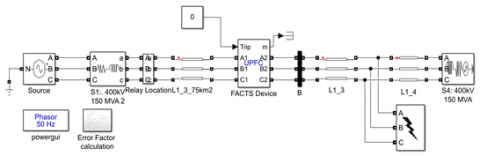Figure 7. A studied system using MATLAB

## 8.png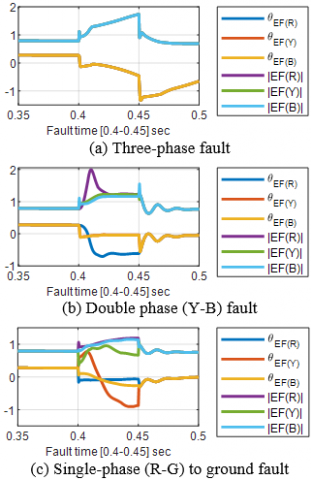Figure 8. The error factor EF when the UPFC changes the power flow from operation point 1 to setpoint 1

## 9.png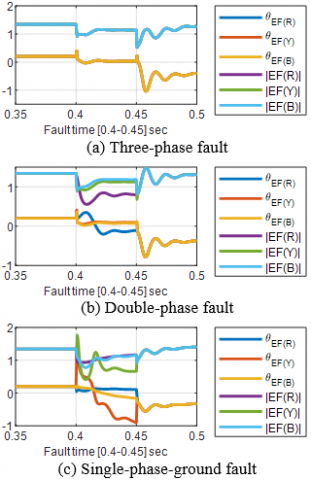Figure 9. The error factor EF when the UPFC changes the power flow from operation point 1 to setpoint 2

5.3 Increase load ability, capacitive mode

In this section, the UPFC is used to increase the transmission line real power from 1 Pu to 1.5 Pu and provide the system with 0.5 Pu reactive power. Figure 10 shows the error factor's magnitude and angle for three fault cases at m=0.75 for operation point (2) and set point (1).

This operation point is very similar to the first scenario (sec. 5.1), but the pre-fault error factor angle is negative in this scenario and positive in the first scenario. This comment is clearly justified in Figures 3 and 5.

## 10.png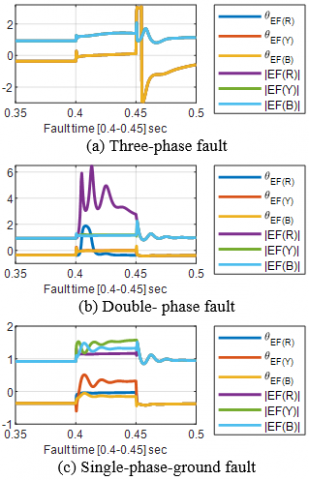Figure 10. The error factor EF when the UPFC changes the power flow from operation point 2 to setpoint 1

5.4 Decrease load ability, capacitive mode

In this section, the UPFC is used to decrease the transmission line real power from 1 Pu to 0.5 Pu and provide the system with 0.5 Pu reactive power. Figure 11 shows the error factor's magnitude and angle for three fault cases at m=0.75 for operation point (2) and set point (2).

## 11.png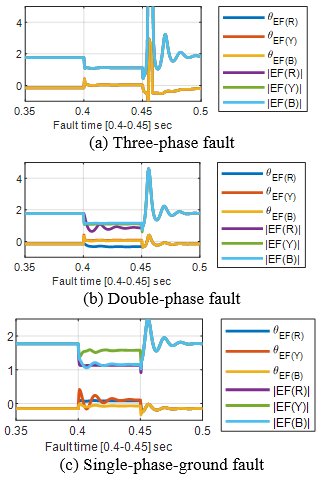Figure 11. EF's magnitude and angle when UPFC changes the power flow from operation point 2 to setting point 2

This operation point is very similar to the second scenario (sec. 5.2), but the pre-fault error factor angle is negative in this scenario and positive in the second scenario. This comment is clearly justified in Figures 3 and 5.

In this section, all the operation conditions of the UPFC are simulated for different faults occurred in the zone one of the compensated transmission lines. From the whole results, it is observed that the error factor before the fault is similar to the previous analysis (shunt and series devices separately). On the other hand, all the cases' error factor is higher than one during the fault. So, it can be generalized that the UPFC compensated transmission line's main problem is the underreach in the distance relay.

6. Conclusion

FACTS devices have tremendous advantages of improving power system stability and regulating the power flow of active and reactive power along the transmission line. Along with this, they can harm the operating characteristics of the protective devices, especially distance protection.

The present paper introduces the effect of the different FACTS devices on the distance relay's performance using the measured impedance between the fault and the distance relay. The impact of FACTS devices on MHO characteristics is presented. The work is evidenced by mathematical analysis and carried out on the two-source test system using MATLAB 2019b Simulink.

This paper provides a comprehensive analysis of the measured impedance in the compensated transmission line. For protection coordinators, UPFC/FACTS controllers, and protection/FACTS researchers, this analysis may be needed.

Appendix

Appendix A: Single Phase fault

Firstly, pre-fault load current ($I_{A L}^{\prime}$) from station A to B (from Figure 2) and voltage ($U_{A L}^{\prime}$) at fault position in phase A can be expressed as:

$I_{A L}^{\prime}=\frac{E_{A}\left(\frac{Z_{1 s h}}{Z_{1 s h}+Z_{1 S A}}\right)-E_{B}}{Z_{1 A}^{\prime}+Z_{1 B}}$$=\frac{\left(\frac{z_{1 s h}}{Z_{1 s h}+Z_{1 S A}}-h e^{-j \delta}\right) E_{A}}{Z_{1 A}^{\prime}+Z_{1 B}} (A1) U_{A L}^{\prime}=E_{A} \frac{Z_{1 s h}}{Z_{1 s h}+Z_{1 S A}}-I_{A L}^{\prime} Z_{1 A} (A2) where: Z_{1 A}^{\prime}=Z_{1 L A}+Z_{1 S E}+\frac{z_{1 S A} Z_{1 S h}}{Z_{1 S A}+Z_{1 S h}}, and Z_{1 B}=Z_{1 L B}+Z_{1 S B} are the positive sequence impedances. When a fault occurs, the impedance of phase A measured at F is: Z_{R}^{\prime}=\frac{U_{A F}}{I_{A F}+3 K_{0} I_{0 A}} (A3) where: U_{A F} faulted phase voltage at A during the fault, I_{A F} fault current measured at A. K_{0} Zero-sequence compensation factor due to remote generators' contribution. I_{0 A} Zerosequence fault current measured at \mathrm{A}. K_{o}^{\prime}=\frac{Z_{o L}-Z_{1 L}}{3 Z_{1 L}} (A4) where, Z_{o L}, Z_{1 L} zero and negative sequence impedance of the faulted line. To calculate I_{A F}, the sequence components are used. The sequence impedances are computed as the Figure A1 below. ## 12.png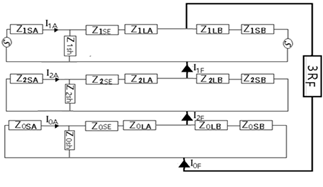Figure A1. Sequence component of a single-phase fault where, positive, negative, and zero equivalent impedances are connected in series in case of single-phase to ground fault. In Transmission lines and generators Z1=Z2. So, the equivalent impedance can be found as flow: Z_{\Delta}^{\prime}=\frac{2 Z_{1 A}^{\prime} Z_{1 B}}{Z_{1 A}^{\prime}+Z_{1 B}}+\frac{Z_{0 A}^{\prime} Z_{0 B}}{Z_{0 A}^{\prime}+Z_{0 B}} (A5) where, Z_{0 A}^{\prime}=Z_{0 L A}+Z_{0 S E}+\frac{z_{0 S A} Z_{0 S h}}{Z_{0 S A}+Z_{0 S h}}, Z_{1 A}^{\prime}=Z_{1 L A}+Z_{1 S E}+$$\frac{z_{1 S A} Z_{1 S h}}{Z_{1 S A}+Z_{1 S h}}, Z_{0 B}=Z_{0 L B}+Z_{0 S B}$ and $Z_{1 B}=Z_{1 L B}+Z_{1 S B}$: The zero and positive sequence impedances. During a single line to ground fault in phase-A, the positive, negative, and zero sequence currents through the fault resistance RF equal:

$I_{1 F}^{\prime}=I_{2 F}^{\prime}=I_{0 F}^{\prime}=\frac{U_{A L}^{\prime}}{Z_{\Delta}^{\prime}+3 R_{F}}$      (A6)

Based on the concept of the current divider, the positive, negative, and zero sequence currents through the relay point (A) equal:

$I_{1 A}^{\prime}=I_{2 A}^{\prime}=C_{1}^{\prime} I_{1 F}^{\prime}=\frac{C_{1}^{\prime} U_{A L}^{\prime}}{Z_{\Delta}^{\prime}+3 R_{F}}$      (A7)

$I_{0 A}^{\prime}=C_{0}^{\prime} I_{1 F}^{\prime}=\frac{C_{0}^{\prime} U_{A L}^{\prime}}{Z_{\Delta}^{\prime}+3 R_{F}}$      (A8)

where, $C_{1}^{\prime}, C_{0}^{\prime}$ are distribution factors and given by:

\begin{aligned} C_{1}^{\prime}=\frac{Z_{1 B}}{Z_{1 B}+Z_{1 A}^{\prime}} & * \frac{Z_{1 s h}}{Z_{1 s h}+Z_{1 S A}}=C_{1}^{\prime \prime} * \frac{Z_{1 s h}}{Z_{1 s h}+Z_{1 S A}} \text { and } C_{0}^{\prime} \\ &=\frac{Z_{0 B}}{Z_{0 B}+Z_{0 A}^{\prime}} * \frac{Z_{0 s h}}{Z_{0 s h}+Z_{0 S A}} \\ &=C_{0}^{\prime \prime} * \frac{Z_{0 s h}}{Z_{0 s h}+Z_{0 S A}} \end{aligned}

Now, the total fault current throw relay at terminal A and fault voltage are given by:

$I_{A F}=I_{A L}^{\prime}+I_{1 A}^{\prime}+I_{2 A}^{\prime}+I_{0 A}^{\prime}=I_{A L}^{\prime}+2 C_{1}^{\prime} I_{1 F}^{\prime}+C_{0}^{\prime} I_{0 F}^{\prime}$      (A9)

\begin{aligned} U_{A F}=\left(I_{1 F}^{\prime}+I_{2 F}^{\prime}\right.& \left.+I_{0 F}^{\prime}\right) R_{F}+\left(I_{1 F}^{\prime} * C_{1}^{\prime \prime}+I_{L A}^{\prime}\right)\left(Z_{1 L A}\right.\\ & \left.+Z_{1 S E}\right)+I_{2 F}^{\prime} * C_{1}^{\prime \prime}\left(Z_{2 L A}+Z_{2 S E}\right) \\ &+I_{0 F}^{\prime} * C_{0}^{\prime \prime}\left(Z_{0 L A}+Z_{0 S E}\right) \end{aligned}     (A10)

$Z_{A}=\frac{U_{A F}}{I_{A F}^{\prime}+3 K_{0} I_{0 A}^{\prime}}$     (A11)

$Z_{A}^{\prime}$$=\frac{3 I_{1 F}^{\prime} R_{F}+\left(I_{1 F}^{\prime} * C_{1}^{\prime \prime}+I_{L A}^{\prime}\right)\left(Z_{1 L A}+Z_{1 S E}\right)+I_{2 F}^{\prime} * C_{1}^{\prime \prime}\left(Z_{2 L A}+Z_{2 S E}\right)+I_{0 F}^{\prime} * C_{0}^{\prime \prime}\left(Z_{0 L A}+Z_{0 S E}\right)}{I_{A L}^{\prime}+2 C_{1}^{\prime} I_{1 F}^{\prime}+C_{0}^{\prime} I_{0 F}^{\prime}+3 K_{0} C_{0}^{\prime} I_{0 F}} (A12) If we ignore the contribution of load current in fault current: Z_{A}^{\prime}$$=\frac{3 R_{F}+\left(2 C_{1}^{\prime \prime}+C_{0}^{\prime \prime}\left(1+3 K_{0}\right)\right)\left(Z_{1 L A}+Z_{1 S E}\right)}{2 C_{1}^{\prime \prime} * \frac{Z_{1 s h}}{Z_{1 s h}+Z_{1 S A}}+(1+3 K)_{0} C_{0}^{\prime \prime} * \frac{Z_{0 s h}}{Z_{0 s h}+Z_{0 S A}}}$     (A13)

$Z_{A}^{\prime} \approx \frac{3 R_{F}}{2 C_{1}^{\prime}+C_{0}^{\prime}\left(1+3 K_{0}\right)}+\left(Z_{1 L A}+Z_{1 S E}\right) \frac{Z_{1 s h}+Z_{1 S A}}{Z_{1 s h}}$      (A14)

Appendix B: Case study parameters

Voltage base: 400 Kv, Power base=100 MW. F=50 Hz.

• Operation point (1):

Source (A): V=1.085 Pu, $\theta=-19.75^{\circ}$, swing. R=8.03, L=0.07875H.

Source (B): V=1.0 Pu, $\theta=-30.8^{\circ}$, swing. R=8.03, L=0.7875 H.

Transmission line (power flow): PL=1 Pu, QL=-0.5 Pu.

• Operation point (2):

Source (A): V=1.17 Pu, $\theta=-20.25^{\circ}$, swing. R=8.03, L=0.07875H.

Source (B): V=0.88 Pu, $\theta=-30.8^{\circ}$, swing. R=8.03, L=0.7875 H.

Transmission line (power flow): PL=1 Pu, QL=0.5 Pu.

• System Parameters:

Transmission line: 150Km, R=0.117 Pu/Km, X=1.547e-3 Pu/Km.

UPFC: Shunt converter parameters: R=0.22/30 Pu, L=0.22 Pu.

UPFC: Series converter parameters: R=0.13/30 Pu, L=0.16 Pu.

UPFC: DC-link: V=40 KV, C=750micro F.

UPFC: Setting point (1) of the UPFC: P=1.5Pu, Q=0,

UPFC: Setting point (2) of the UPFC: P=0.5 Pu, Q=0.

References

 Sadhu, R., Bhowmik, P.S. (2017). Different setting of unified power flow controller (UPFC) and its effect on performance of distance relay. In: Chattopadhyay S., Roy T., Sengupta S., Berger-Vachon C. (eds) Modelling and Simulation in Science, Technology and Engineering Mathematics. MS-17 2017. Advances in Intelligent Systems and Computing, 749: 179-190. Springer, Cham. https://doi.org/10.1007/978-3-319-74808-5_16

 Guan, R., Xue, Y., Zhang, X.P. (2018). Advanced RTDS-based studies of the impact of STATCOM on feeder distance protection. The Journal of Engineering, 2018(15): 1038-1042.‏ http://doi.org/10.1049/joe.2018.0179

 Zhang, W.H., Lee, S.J., Choi, M.S., Oda, S. (2010). Considerations on distance relay setting for a transmission line with STATCOM. IEEE Power and Energy Society General Meeting, pp. 1-5. http://doi.org/10.1109/PES.2010.5588192

 Aboelazm Y.M., Wahba W.E., Moustafa Hassan, M.A. (2018). Simulation of advanced STATCOM for voltage swell mitigation in large-scale test system based on swarm intelligence algorithms, European Journal of Electrical Engineering, 20(3): 253-266. https://doi.org/10.3166/EJEE.20.253-266

 Sidhu, T.S., Varma, R.K., Gangadharan, P.K., Albasri, F.A., Ortiz, G.R. (2005). Performance of distance relays on shunt-FACTS compensated transmission lines. IEEE Transactions on Power Delivery, 20(3): 1837-1845. https://doi.org/10.1109/TPWRD.2005.848641

 Albasri, F.A., Sidhu, T.S., Varma, R.K. (2007). Performance comparison of distance protection schemes for shunt-FACTS compensated transmission lines. IEEE Trans. Power Delivery, 22(4): 2116-2125. https://doi.org/10.1109/TPWRD.2007.900283

 Said, B.M., Eddine, K.D., Salim, C. (2020). Artificial neuron network based faults detection and localization in the high voltage transmission lines with Mho distance relay. Journal Européen des Systèmes Automatisés, 53(1): 137-147. https://doi.org/10.18280/jesa.530117

 Kazemi, A., Jamali, S., Shateri, H. (2009). Comparing impacts of SSSC and STATCOM on measured impedance at relaying point. 2009 IEEE Power & Energy Society General Meeting, pp. 1-7. https://doi.org/10.1109/PES.2009.5275478

 Abdollahzadeh, H., Mozafari, B., Tavighi, A., Marti, J. (2013). Impact of shunt capacitance of an SSSC compensated transmission line on the performance of distance relays. 2013 IEEE Power and Energy Society General Meeting, pp. 1-5. https://doi.org/10.1109/PESMG.2013.6672807

 Khederzadeh, M., Ghorbani, A., Salemnia, A. (2009). Impact of SSSC on the digital distance relaying. IEEE Power and Energy Society General Meeting, pp. 1-8. https://doi.org/10.1109/PES.2009.5275271

 Alwan, S.H. (2019). Protection of transmission line based on the severity index using generation rescheduling strategy. European Journal of Electrical Engineering, 21(6): 523-530. https://doi.org/10.18280/ejee.210606

 Sun, Z.L., Lv, G., Luo, Z.Y., Xie, C.Y., Wang, W. (2019). A novel automatic detection model for single line-to-ground fault. Journal Européen des Systèmes Automatisés, 52(3): 229-233. https://doi.org/10.18280/jesa.520302

 Khederzadeh, M. (2008). UPFC operating characteristics impact transmission line distance protection. 2008 IEEE Power and Energy Society General Meeting - Conversion and Delivery of Electrical Energy in the 21st Century, pp. 1-6. https://doi.org/10.1109/PES.2008.4596219

 Jamali, S., Kazemi, A., Shateri, H. (2009). Effects of UPFC on measured impedance by distance relay in double-circuit lines. 2009 IEEE Power & Energy Society General Meeting, pp. 1-8. https://doi.org/10.1109/PES.2009.5275344

 Jamali, S., Kazemi, A., Shateri, H. (2010). Comparing series and shunt reactive power compensation via UPFC from distance relay point of view. Transmission and Distribution Conference and Exposition, pp. 1-6. https://doi.org/10.1109/TDC.2010.5484345

 Hlngoranl, N.G., Gyugyl, L. (2000). Understanding FACTS Concepts and Technology of Flexible AC Transmission Systems. Wiley-IEEE Press.

 Xia, Y.Q., Li, K.K., David, A.K. (1994). Adaptive relay setting for stand-alone digital distance protection. IEEE Transactions on Power Delivery, 9(1): 480-491.‏ https://doi.org/10.1109/61.277720

 Sharma, R.S., Dewan, L., Chatterji, S. (2019). Fault analysis through Euclidean distance classifier in CSTR. Modelling, Measurement and Control A, 92(1): 7-13. https://doi.org/10.18280/mmc_a.920102

 De Andrade, V., Sorrentino, E. (2010). Typical expected values of the fault resistance in power systems. 2010 IEEE/PES Transmission and Distribution Conference and Exposition: Latin America (T&D-LA), pp. 602-609.‏ https://doi.org/10.1109/TDC-LA.2010.5762944

 Almomani, M., Algharaibeh, S.F. (2020). Modelling and Testing of a numerical pilot distance relay for compensated transmission lines. International Journal of Scientific Research and Engineering Development, 3(6): 776-786.